# Data Preprocessing in Data Science

## Data Preprocessing in Data Science with Examples

In this article, I am going to discuss Data Preprocessing in Data Science with Examples. Please read our previous article where we discussed Feature Selection Case Study in Data Science with Examples.

##### Data Preprocessing in Data Science

Data preprocessing refers to the steps involved in transforming or encoding data so that it may be easily interpreted by a computer. The algorithm must be able to quickly interpret the data’s attributes in order for a model to be accurate and exact in predictions.

Due to their various origins, the majority of real-world datasets are particularly prone to missing, inconsistent, and noisy data. Applying data mining algorithms to this noisy data would produce poor results since they would be unable to detect patterns. As a result, data preprocessing is critical for improving overall data quality.

There are 4 major stages of data preprocessing –

1. Data Cleaning
2. Data Integration
3. Data Transformation
4. Data Quality Assessment
###### Data cleaning –

It is a step in the data preprocessing process that involves filling in missing values, smoothing noisy data, resolving inconsistencies, and removing outliers.

###### Data integration –

It is a data preparation phase that combines data from numerous sources into a single larger data storage, such as a data warehouse.

###### Data transformation –

It is a technique for converting high-quality data into different formats by altering the value, structure, or format of data using techniques such as scaling, normalization, and others.

###### Data Quality Assessment –

It refers to the statistical procedures that must be followed to ensure that the data is free of errors and hence of good quality.

##### How to Preprocess Your Data?

Data preprocessing is a crucial stage in Machine Learning because the quality of data and the relevant information that can be gleaned from it has a direct impact on our model’s capacity to learn; consequently, preprocessing our data before feeding it into our model is critical.

1. Handling Null Values
2. Handling Categorical Variables
3. Multicollinearity
4. Feature Scaling
##### Handling Null Values –

There are usually a few null values in any real-world dataset. Regardless of whether the problem is a regression, classification, or any other type, no model can handle NULL or NaN variables on its own, thus we must intervene. NaN is used to representing NULL in Python. So don’t get them mixed up; they can both be used interchangeably. First and foremost, we must determine whether or not our dataset contains null values. The isnull() method can be used to do this.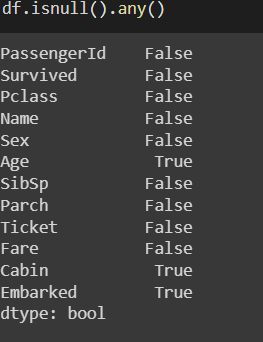To check total null values in every column, you can use the isnull().sum() method.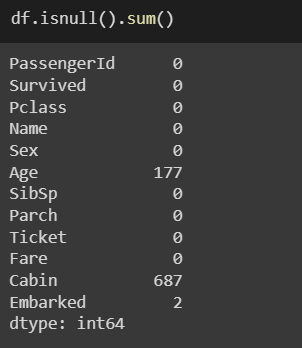We have a number of options for dealing with this issue.

##### a. Dropping the rows or columns with null values –
1. dropna() can be used for dropping rows/columns with null values. It accepts several options –
2. axis – axis=0 for removing rows and axis=1 for removing columns.
3. thresh – Sets the threshold value; for example, if thresh=5, all rows with fewer than 5 real values would be removed.
4. inplace – Your data frame will not be changed by default. In order for these modifications to be reflected in your data frame, you must use inplace = True.

However, removing the rows and columns from our dataset is not the ideal approach because it can result in severe data loss. If you have 300K data points, eliminating 2–3 rows won’t have much of an impact on your dataset; however, if you just have 100 data points, and 20 of them contain NaN values for a particular field, you won’t be able to simply eliminate those rows.

##### b. Handling features will numerical values –

We can use the fillna() method for filling rows with null values.

1. If the missing data is a numerical variable, the mean or median value is used to fill it in.
2. Filling in the numerical value with 0 or -999, or a number that will not appear in the data. This can be done in order for the system to notice that the data isn’t real or isn’t the same.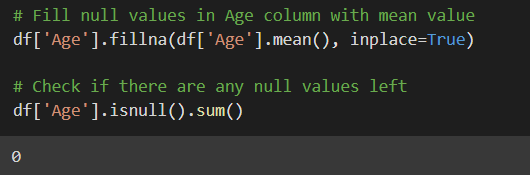##### c. Handling features will categorical values –

For the missing values, a new type can be added to the categorical value. We can also use the mode value (most occurring value) to fill in the null categorical value.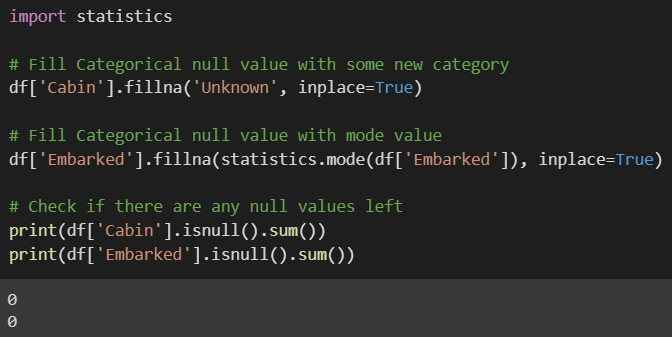##### Handling Categorical Variables –

Handling categorical variables is another key part of Machine Learning. Categorical variables are variables that are discrete rather than continuous in nature. Because algorithms interpret numerical values rather than categorical values, we must ensure that such characteristics are provided in the form of numerical data. We can accomplish this in two ways:

##### 1. Label Encoding –

For categorical variables, label encoding is a popular encoding approach. Each label is given a unique integer based on alphabetical order in this technique. The scikit-learn library can be used to implement Label Encoding.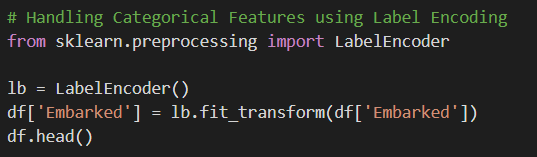##### 2. One Hot Encoding –

Another typical technique for dealing with categorical information is one-hot encoding. It simply adds more characteristics to the categorical feature dependent on the number of unique values. Every category’s unique value will be added as a feature. One-Hot Encoding is the method of producing dummy variables.

```# Handling Categorical Features using One Hot Encoding
dummy = pd.get_dummies(df.Sex, prefix='Gender')
```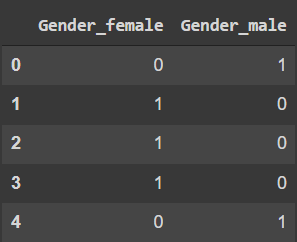```# Merge the two dataframes
df = pd.merge(left=df, right=dummy, left_index=True, right_index=True)

# Let's drop rest of the columns now
df.drop(['Sex','Gender_male'], axis=1, inplace=True)
```
##### Multicollinearity

When we have features that are highly reliant on one other, we have multicollinearity in our dataset. We won’t be able to utilize our weight vector to calculate feature importance if our dataset has multicollinearity. The interpretability of our model is influenced by multicollinearity. By removing rows with a high correlation, multicollinearity is avoided.

##### Feature Scaling

Feature scaling is a technique for normalizing a set of independent variables or data components. It is also known as data normalization or standardization in data processing and is usually done to make features comparable.

##### Feature Scaling

Feature scaling is a technique for normalizing a set of independent variables or data components. It is also known as data normalization in data processing and is usually done during the data preprocessing step.

For instance, if you have multiple independent variables such as age, salary, and height, with ranges of (18–100 Years), (25,000–75,000 Euros), and (1–2 Meters), feature scaling would help them all be in the same range, for example, centered around 0 or in the range (0,1) depending on the scaling technique.

Let’s have a look at the various ways of feature scaling. The following are the most popular approaches available:

1. Normalization
2. Standardization
##### Normalization –

The simplest method, also known as min-max scaling or min-max normalization, consists of rescaling the range of features to scale the range in [0, 1]. The general normalization formula is as follows: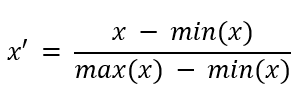The maximum and minimum values of the feature are represented by max(x) and min(x), respectively.

##### Standardization –

Feature standardization reduces the variance and means of each feature in the data to zero. The usual technique of computation is to find the distribution mean and standard deviation for each feature and then use the following formula to calculate the new data point: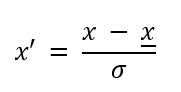Here, is the feature vector’s standard deviation and is the feature vector’s average.

##### Standardization vs Normalization

When the data distribution does not follow a Gaussian distribution, normalization is useful. Normalization gets highly impacted by outliers.

When the data has a Gaussian distribution, standardization can be beneficial. This, however, does not have to be the case. Standardization does not have a bounding range, thus even if there are outliers in the data, standardization won’t get affected by them.

##### Feature Scaling Case Study

The Titanic’s sinking is one of the most well-known shipwrecks in history. While survival required some luck, it appears that some groups of people were more likely to live than others. The dataset of passengers and their survival history may be found here.

Let’s see how we can preprocess this dataset and scale its features to make them ready to be fed in ML algorithms.

```# Import Necessary Libraries
import numpy as np
import pandas as pd
import matplotlib.pyplot as plt
import seaborn as sns
# Import dataset
```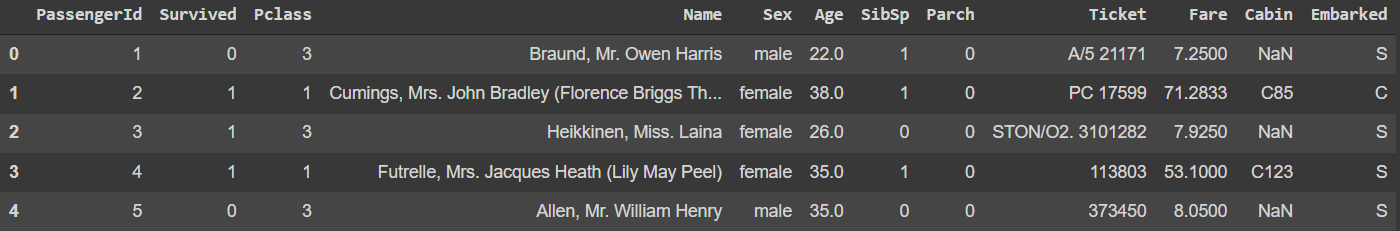```# Check information of dataset
df.info()
```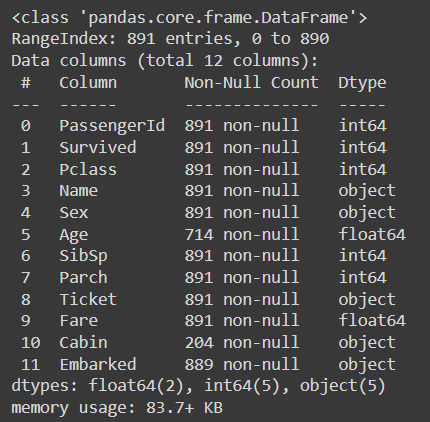```# Check presence of null values
df.isnull().sum()
```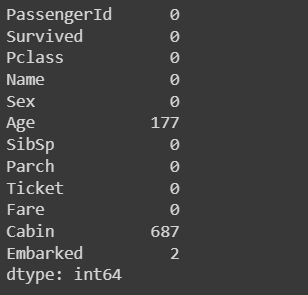```# Fill null values in Age column with mean value
df['Age'].fillna(df['Age'].mean(), inplace=True)

# Check if there are any null values left
df['Age'].isnull().sum()
import statistics

# Fill Categorical null value with some new category
df['Cabin'].fillna('Unknown', inplace=True)

# Fill Categorical null value with mode value
df['Embarked'].fillna(statistics.mode(df['Embarked']), inplace=True)

# Handling Categorical Features using Label Encoding
from sklearn.preprocessing import LabelEncoder

lb = LabelEncoder()
df['Embarked'] = lb.fit_transform(df['Embarked'])
```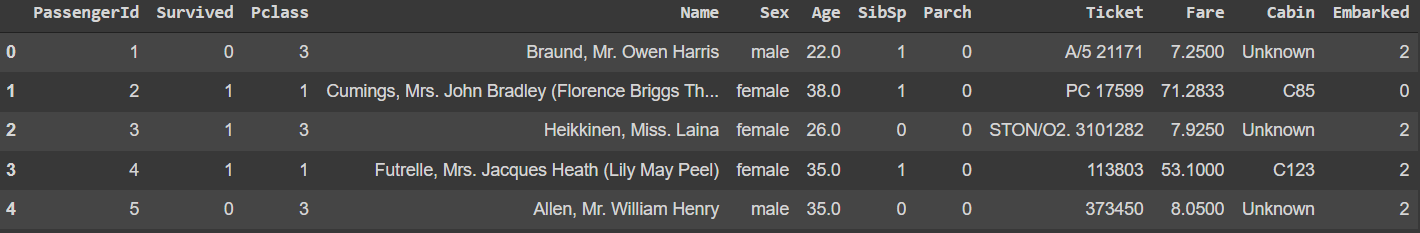```# Handling Categorical Features using One Hot Encoding
dummy = pd.get_dummies(df.Sex, prefix='Gender')
```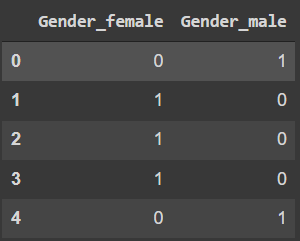```# Merge the two dataframes
df = pd.merge(left=df, right=dummy, left_index=True, right_index=True)

# Let's drop rest of the columns now
df.drop(['Sex','Gender_male'], axis=1, inplace=True)
```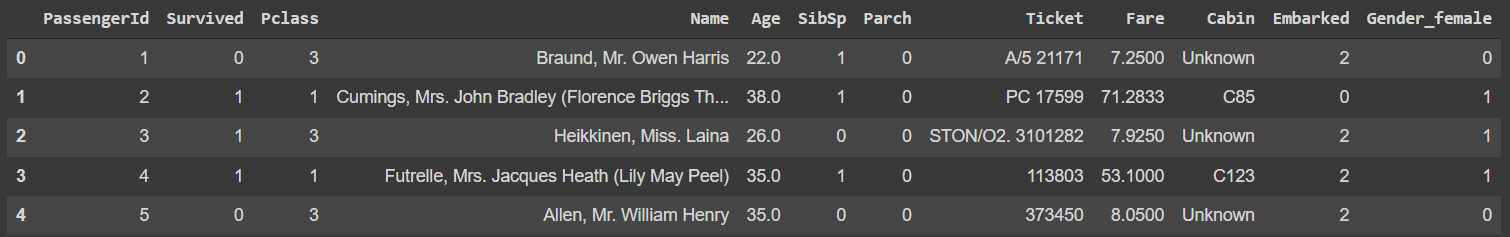```# Let's drop all the unnecessary features now
df.drop(['PassengerId','Name', 'Ticket', 'Cabin'], axis=1, inplace=True)

# Feature Scaling
# First Splitting Independent and target features
y = df.pop('Survived')
x = df

# Normalization
# data normalization with sklearn
from sklearn.preprocessing import MinMaxScaler

# fit scaler
norm = MinMaxScaler().fit_transform(x)
norm
```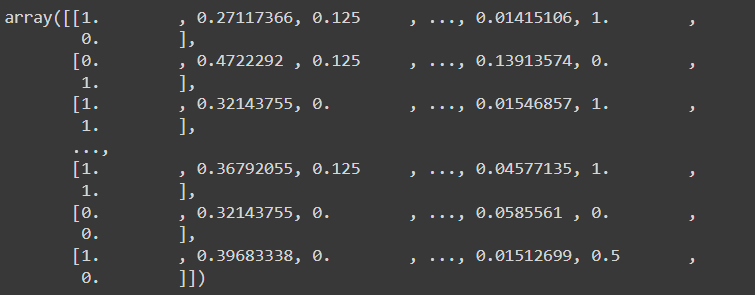```# Standardization
# data standardization with sklearn
from sklearn.preprocessing import StandardScaler

# fit scaler
sc = StandardScaler().fit_transform(x)
sc
```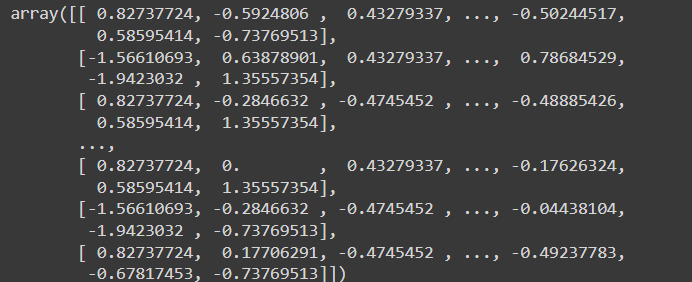In the next article, I am going to discuss Data Preprocessing in Data Science with Examples. Here, in this article, I try to explain Data Preprocessing in Data Science with Examples. I hope you enjoy this Data Preprocessing in Data Science with Examples article.